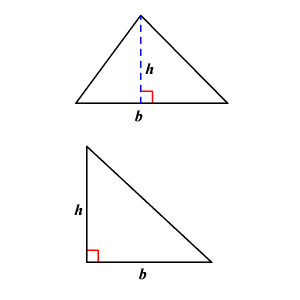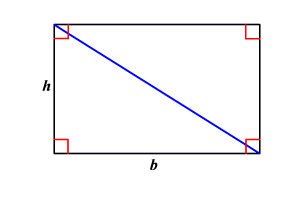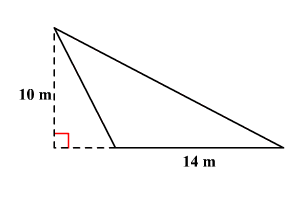# Triangles: Area

A triangle is a polygon with three sides.We know that the area of a rectangle is $b×h$ , where $b$ is the base and $h$ is the height of the rectangle. Using the formula for the area of a rectangle, we can find the area of a triangle.A diagonal of a rectangle separates the rectangle into two congruent triangles. The area of each triangle is one-half the area of the rectangle.

So, the area $A$ of a triangle is given by the formula $A=\frac{1}{2}bh$ where $b$ is the base and $h$ is the height of the triangle.

Example:

Find the area of the triangle.The area $A$ of a triangle is given by the formula $A=\frac{1}{2}bh$ where $b$ is the base and $h$ is the height of the triangle.

Substitute $14$ for $b$ and $10$ for $h$ in the formula.

$A=\frac{1}{2}\left(14\right)\left(10\right)$

Simplify.

$\begin{array}{l}A=\frac{1}{2}\left(140\right)\\ \text{\hspace{0.17em}}\text{\hspace{0.17em}}\text{\hspace{0.17em}}\text{\hspace{0.17em}}\text{\hspace{0.17em}}=70\end{array}$

The area of the triangle is $70$ square meters.